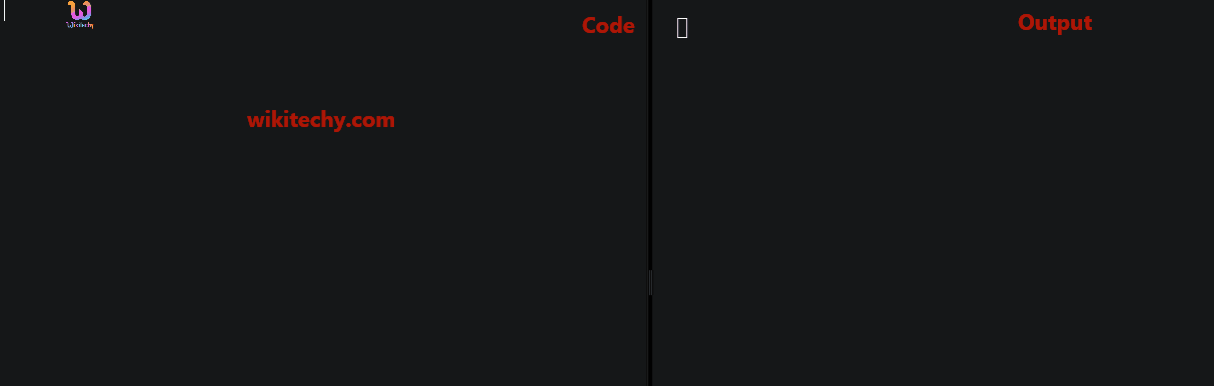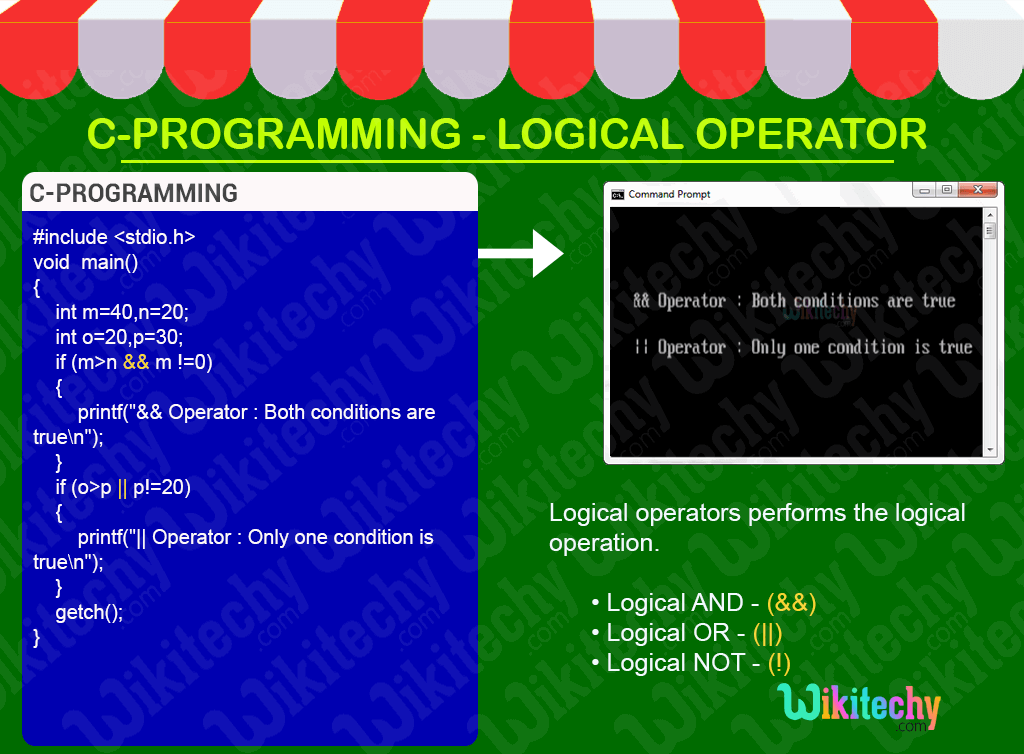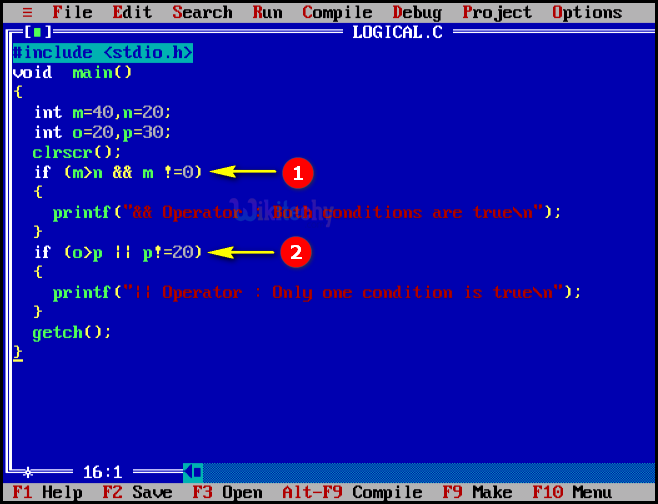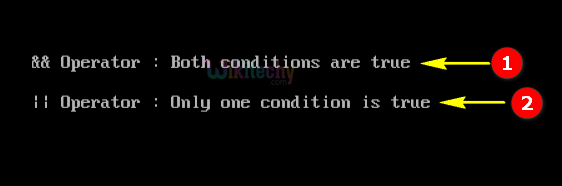# C - Logical OperatorsLearn C - C tutorial - C logical operators - C examples - C programs

## C Logical Operators - Definition and Usage

• In C-Programming the logical operators are used for defining the logical operation.
• Generally, there are three logical operators in c language.
• logical AND (&&)
• logical OR (||)
• logical NOT (!)Operators Name Example Description
&& logical AND (x>5)&&(y<5) It returns true when both conditions are true
|| logical OR (x>=10)||(y>=10) It returns true when atleast one of the condition is true
! logical NOT !((x>5)&&(y<5)) It reverses the state of the operand “((x>5) && (y<5))”If “((x<5) && (y<5))” is true, logical NOT operator makes it false

## C Code - Explanation1. In this statement we are using the logical operator AND(&&) if both conditions are true. Here m>n condition will be satisfied because m=40 and n=20 and the next condition is that m not equals to “0” is satisfied so the print statement prints the output as “&& operator: Both condition is true” .
2. In this statement we are using the logical operator OR (||) so only one condition is satisfied. Here o>p condition will not be satisfied because o=20 and p=30 and also the next condition “m” is not equals to “20” so the print statement prints the output as “||operator: only one condition is true” .

## Sample Output - Programming Examples1. Here in this output the “if” condition satisfy both condition so output will be displayed as “&& Operator: Both conditions are true” in the output window.
2. Here in this output the “if” condition satisfy only one condition so output will be displayed as “|| Operator: Only one condition is true” in the output window.